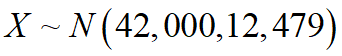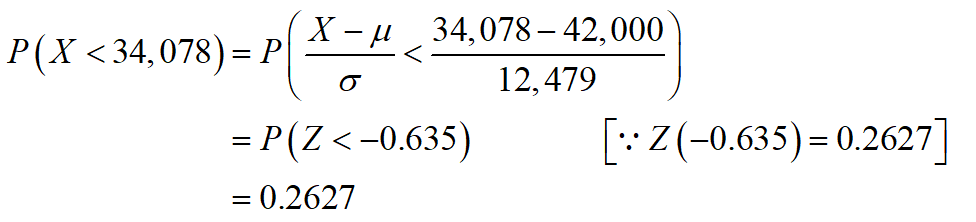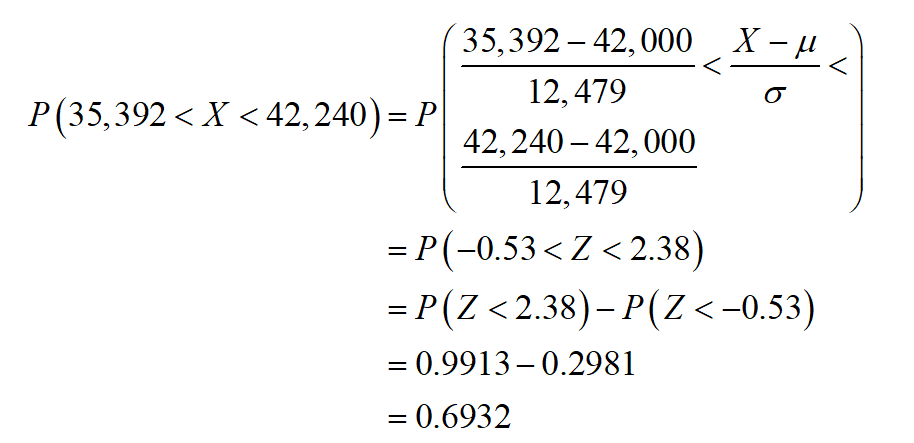# The number of ants per acre in the forest is normally distributed with mean 42,000 and standard deviation 12,479. Let X = number of ants in a randomly selected acre of the forest. Round all answers to 4 decimal places where possible.a. What is the distribution of X? X ~ Nb. Find the probability that a randomly selected acre in the forest has fewer than 34,078 ants.c. Find the probability that a randomly selected acre has between 35,392 and 42,240 ants. d. Find the first quartile.

Question
12 views

The number of ants per acre in the forest is normally distributed with mean 42,000 and standard deviation 12,479. Let X = number of ants in a randomly selected acre of the forest. Round all answers to 4 decimal places where possible.

a. What is the distribution of X? X ~ N

b. Find the probability that a randomly selected acre in the forest has fewer than 34,078 ants.

c. Find the probability that a randomly selected acre has between 35,392 and 42,240 ants.

d. Find the first quartile.

check_circle

Step 1

a.

The distribution of X,Step 2

b.

The probability that a randomly selected acre in the forest has fewer than 34,078 ants is,Thus, the probability that a randomly selected acre in the forest has fewer than 34,078 ants is 0.2627.

Step 3

c.

The probability that a randomly selected acre in the forest has between 35,392 and 42,240 ants is,Thus, the probability that a randomly selected acre in the forest has between 35,392 and 42,240 ants is 0.6932.

...

### Want to see the full answer?

See Solution

#### Want to see this answer and more?

Solutions are written by subject experts who are available 24/7. Questions are typically answered within 1 hour.*

See Solution
*Response times may vary by subject and question.
Tagged in

### Statistics